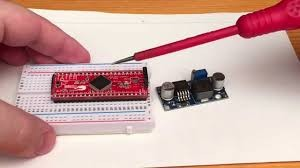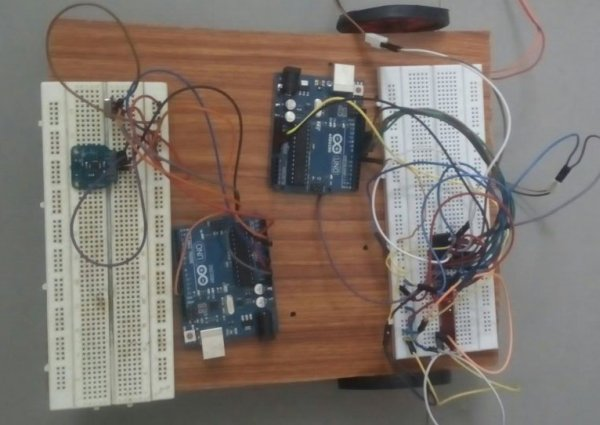# How to control LM2596 buck-converter with microcontroller

Every now and then someone asks on different forums if there is an way to control cheap LM2596 modules with an Arduino or another microcontroller. I decided to demonstrate one solution that might be basic electronics for some, but still many don’t know about.

Those buck converters will change the output voltage to make the feedback pin, connected to the output via a voltage divider, become 1.25V or so. If feedback is higher, output gets lower and vice versa. If one changes the ratio of resistors in voltage divider, output voltage will change. This is usually done by turning a trimmer resistor with a screwdriver. That is good enough for many applications where voltage will be set only once, but sometimes there is a need to adjust the output voltage more frequently.External voltage can pull the feedback pin’s voltage higher or lower when applied to it through a resistor. That would make a summer circuit where output voltage of buck converter and external voltage are inputs and output is junction which connects to the feedback pin connects. That external voltage can be generated with just about any microcontroller. Microcontroller generates PWM signal, which is smoothed with RC low pass filter and then buffered with an operational amplifier configured as a voltage follower. Thanks to Hackaday’s Al Williams for pointing this out: I didn’t remember to mention this clearly enough in the video: Circuit shown in this demonstration needs to have really stable power supply for the microcontroller, as the PWM signal’s amplitude depends on the power supply. So any changes on power supply’s voltage will affect the amplitude of the generated voltage and therefore the buck-converter’s output voltage.

In my demonstration the PWM-signal’s maximum voltage and therefore maximum signal generated is 5V. When buck converter’s maximum output is set high enough (lets say 12->V) adjustable resistor’s (which is one between output and feedback) resistance will be relative high compared to the resistance of buck converter module’s fixed resistor (which is 330ohm in this case). Therefore when trying to adjust converter’s output to zero or as low as possible with microcontroller, the output voltage’s effect to voltage on feedback pin will be marginal. Therefore we just need to figure out resistor for voltage divider that divides 5V to 1.25V, where lower resistor is 330 ohm. That is simple task: R = (5V * 330ohm – 1.25V * 330ohm)/1.25V = 990ohm

For More Details: How to control LM2596 buck-converter with microcontroller

#### This Post / Project can also be found using search terms:

• controll lm2596 microcontroller
• lm2596 arduino controlled